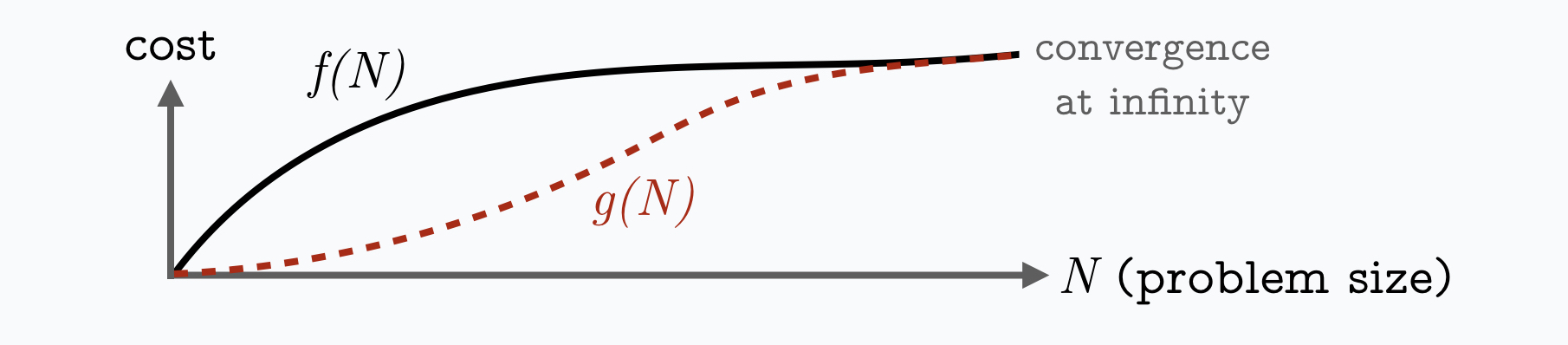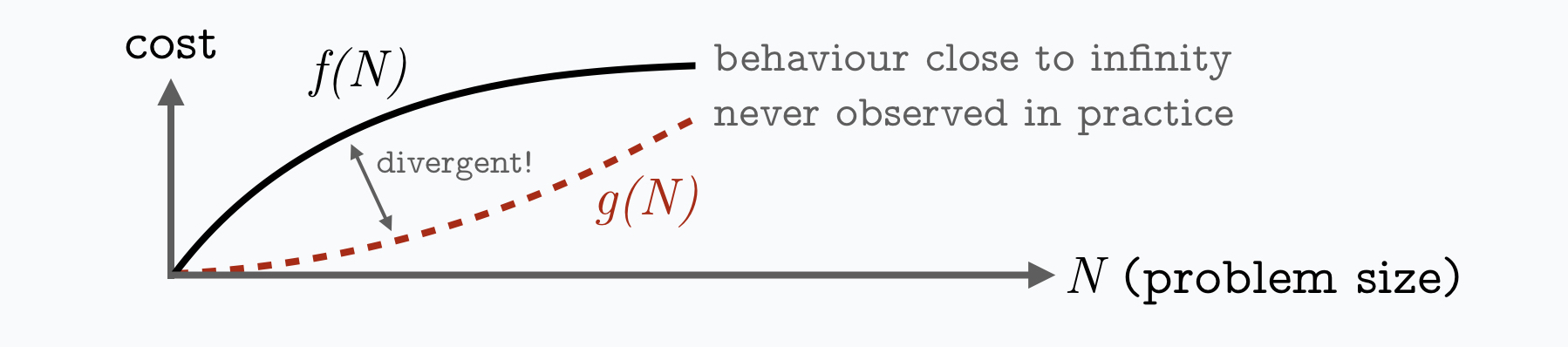### Predictive theory

Theory with predictive power is the backbone of natural sciences, yet it has received far less attention in computer science where theory is often only loosely connected to real-world behaviour.

#### Asymptotic Computational ComplexitySuppose, the function f(N) describes the time an algorithm needs to process N data elements on a certain computer. Horizontal asymptotes describe the behaviour of the function's curve at negative and positive infinity. We can find a function g(N) to describe the asymptotes by omitting elements of f(N) that become irrelevant for N approaching infinity, such that the functions f and g behave the same way at infinity. Computer scientists then write in Big-O notation either O(g(N)) or Θ(g(N)) as the asymptotic complexity of the algorithm (the former indicates an upper bound, the latter a tight bound).

#### When Asymptotic Analysis FailsSome simple facts about asymptotic analysis that too often are not taught in the class room:
• Hidden function terms: Asymptotic complexities do not just hide constants, but any terms that happen to become irrelevant at infinity!
• Heuristic: Asymptotic complexities can be an arbitrarily poor approximation for all problem instances that could occur in the real-world!
• Misleading Comparisons: An approach with inferior asymptotic complexity, can be vastly superior for any practically relevant problem instances.
• Overlooked: While average-case, parametrised and smoothed complexity were devised to address the shortcomings of worst-case analysis, the shortcomings of asymptotic analysis in general are mostly overlooked.
Going back to the example of a cost function f and an asymptotic approximation g for a problem size N. Practically, an algorithm is never executed for astronomically large values of N and it tells us very little about the behaviour of the algorithm for finite values of N, unless f(N) happens to be very similar to g(N). This applies not only to worst-case, but also to average-case and smoothed complexities that all presume N to be infinitely large. So, why are complexities even useful? One original motivation, was to obtain something that remains the same, even if we presume our hardware to be twice or ten times as fast. It is also typically a lot easier to derive and interpret than the original function f, but generally speaking knowing f(N) is of course better. Somehow this reality got lost in some corners of the theoretical computer science community and asymptotic complexity is surprisingly often misunderstood as having predictive power. While this might be true for some very simple algorithms where the asymptotic complexity is very similar to the actual formula, this does not generally hold. This does not mean that asymptotic analysis needs to be abandoned, but it needs to be employed with some care and when it fails some alternatives need be devised. The motivation of hardware independence is also not as crucial for space complexities, as one can either use words or bits as universal units across different types of hardware.

#### Examples

A simple example are range trees, an index structure for geometric data whose complexities are polynomials of logarithms for probing times and space overhead. Any polynomials of logarithms (with an arbitrary large constant degree) are at infinity better than sub-linear functions such as square root N. Yet, it is easy to demonstrate that range trees are not useful beyond three or maybe four data dimensions. Meanwhile, an index structure with a complexity equal to square root N could of course be very practical. The asymptotic complexities on their own do not reveal which one will be practical or not.

#### Beyond Asymptotic Analysis: How to get Predictive Power

If the actual function f defined in the previous section is known, then it can be evaluated for various parameters to compare it to other functions in order to predict if one approach is expected to perform better than the other. If deriving f(N) is too difficult, one can at least try to approximate it by embedding the asymptotic complexity into a linear function or polynomial that is fitted to match the known values of f(N) based on empirical measurements. If f(N) is data-dependent, one can either perform a (non-asymptotic) worst-case analysis or obtain the average-case performance for some stochastic model of the data. The theoretical predictions can then be evaluated against empirical measurements.